## 20 Questions MCQ Test Mathematics (Maths) Class 6 | Olympiad Test: Data Handling

Description
Attempt Olympiad Test: Data Handling | 20 questions in 20 minutes | Mock test for Class 6 preparation | Free important questions MCQ to study Mathematics (Maths) Class 6 for Class 6 Exam | Download free PDF with solutions
QUESTION: 1

### The line plot below shows how students scored on last week's maths test.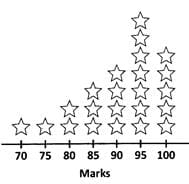Key: Each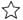= 1 student How many students scored 95 or higher on the test?

Solution:

7+5 = 12 students

QUESTION: 2

### If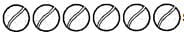stands for 30, how much does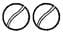stand for?

Solution:

Each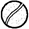stands for  30/6 = 5 So,stands for 10.

QUESTION: 3

### Direction: Question is based on this table and pictograph which show the number of school teachers in some cities.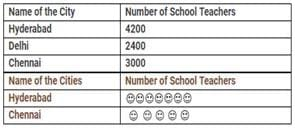How many school teachers does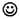represent?

Solution:

Here, Hyderabad has 4200 school teachers, and they are represneted by 7 images.

Therefore= 4200 / 7 = 600

QUESTION: 4

Question is based on this table and pictograph which show the number of school teachers in some cities.Which of the following is the correct representation of the number of school teachers in Delhi?

Solution:

Eachrepresents 600. So, 2400 can be represented by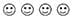QUESTION: 5

If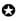represents 5 balloons, number of symbols to be drawn to represent 60 balloons is

Solution:= 5 balloons

60 balloons will be represented = 60/5 = 12 symbols

QUESTION: 6

Direction: The pictograph given shows the number of letters were collected from a post box on each day of a certain week.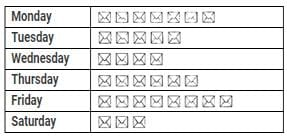Key: Each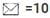letters On which day were the minimum number of letters collected?

Solution:

According to the given pictograph, minimum number of letters were collected on Saturday (i.e.,)
3 × 10 = 30 letters.

QUESTION: 7

The pictograph given shows the number of letters were collected from a post box on each day of a certain week.Key: Eachletters What is the total number of letters collected from the post box over the whole week?

Solution:

Total no. of letters collected in the whole week = 33 Given eachletters
∴ Total no. of letters
= 33 × 10
= 330 letters.

QUESTION: 8

The pictograph given shows the number of letters were collected from a post box on each day of a certain week.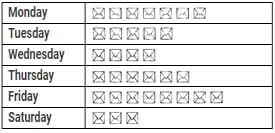Key: Each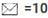letters How many more letters were collected on Friday than on Wednesday?

Solution:

No. of letters collected on Friday = 8×10 = 80 letters
No. of letters collected on Wednesday = 4 × 10 = 40  letters
Difference = (80−40) = 40 letters

QUESTION: 9

If O represents 5 eggs how many eggs does OOOO represent?

Solution:

O = 5 eggs

OOOO = 5 x 4 = 20 eggs

QUESTION: 10

If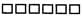stands for 300, what doc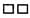stand for?

Solution:

Ifstands for 300 then stands for 50. So,stand for 2 × 50 =100.

QUESTION: 11

Direction: Question is based on this table and pictograph which show the number of government employees in some places.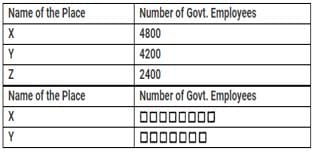How many employees does a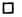represent?

Solution:

Here, X place has 4800 employees, and they are represneted by 8 images.

Therefore= 4800 / 8 = 600

QUESTION: 12

Question is based on this table and pictograph which show the number of government employees in some places.Which of the following is the correct representation of the number of employees in Z?

Solution:

Here, X place has 4800 employees, and they are represneted by 8 images.

Therefore 1 image = 4800 / 8 = 600

Number of employees at Z = 2400

Number of symbols needed to represent Z = 2400 / 600 = 4

QUESTION: 13

What is the number of houses represented by the figure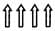if each symbol represents 15 houses?

Solution:

Each symbole represents 15 houses.

Therefore= 4 x 15 = 60

QUESTION: 14

A symbol is used to represent 100 flowers. How many symbols are to be drawn to show 800 flowers?

Solution:

One symbol = 100 flowers

800 flowers = 800 / 100 = 8 symbols

QUESTION: 15

Direction: Answer the following set of questions by reading the pictograph given.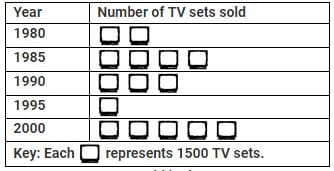How many TV sets are sold in the year 1995?

Solution:

In the pictograph, one image equals 1500 TV sets.

Total number of TV sets sold in the year 1995 were 1 x 1500 = 1500

QUESTION: 16

Direction: Answer the following set of questions by reading the pictograph given.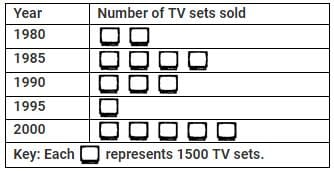What is the number of TV sets sold in the year 1980?

Solution:

In the pictograph, one image equals 1500 TV sets.

Total number of TV sets sold in the year 1980 were 2 x 1500 = 3000

QUESTION: 17

Direction: Answer the following set of questions by reading the pictograph given.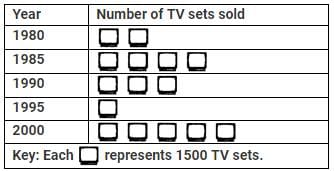In which year were 4500 TV sets sold?

Solution:

In the pictograph, one image equals 1500 TV sets.

In the year 1990, 3 x 1500 = 4500 TV sets were sold.

QUESTION: 18

If one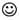symbol represents 10 children, how many children are represented by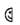Solution:

If= 10 children

Then,= Half of 10 = 5 Children

QUESTION: 19

What is a graph drawn using vertical bars called?

Solution:

A graph drawn using vertical bars is called a bar graph.

The bar graphs can be either vertical or horizontal.

QUESTION: 20

If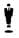represents 12 men then how many men does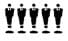represent?

Solution:

If= 12 men

Then,= 12 x 5 = 60 menUse Code STAYHOME200 and get INR 200 additional OFF Use Coupon Code